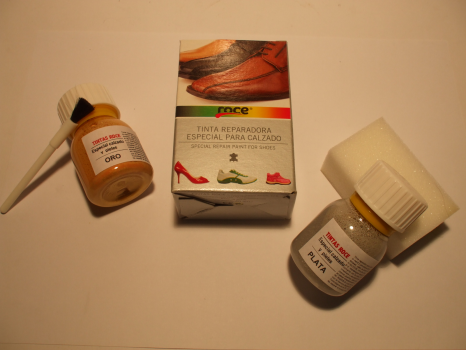TINTA ROCE 40 MLReferência:T030Adicionar ao carrinho
Mais informação

## Características do Produto

TINTA A BASE AGUA COM GRANDE RESISTENCIA E ACABAMENTO NATURAL.
AMPLA GAMA DE CORES.
FRASCO  40MLTANGÓN (Ref. 01) DESIERTO (Ref.05) VAINILLA (Ref. 09) ÁMBAR (Ref. 13) LILA (Ref. 17)LINEN (Ref. 02) SALINAS (Ref. 06) CIELO (Ref. 10) CELESTE (Ref. 14) MAR (Ref. 18)BEIG (Ref. 03) ARENA (Ref. 07) BLANCO (Ref. 11) NUBOL (Ref. 15) FIORDO (Ref. 19)CAMEL (Ref. 04) NÉCTAR (Ref.08) OLIMPO (Ref. 12) AQUA (Ref. 16) TERRANO (Ref. 20)AZULÓN (Ref. 21) PISTACHO (Ref.25) NARANJA (Ref. 29) FUXIA (Ref. 33) GUINDA (Ref. 37)TRÉBOL (Ref.22) MELÓN (Ref. 26) MANDARINA(Ref.30) CHICLE (Ref. 34) RIOJA (Ref. 38)MANZANA (Ref. 23) CLOROFILA(Ref.27) ROSA (Ref. 31) ROJO (Ref. 35) BURDEOS (Ref. 39)KIWI (Ref. 24) SOL (Ref. 28) QUISQUILLA (Ref.32) RUBÍ (Ref. 36) MIEL (Ref.40)TURQUESA (Ref.41) BRANDY (Ref. 45) FORENS (Ref. 49) NAVY (Ref. 53) ORO (Ref. 57)COÑAC (Ref. 42) CACAO (Ref. 46) KAKI (Ref. 50) AZUL (Ref. 54) PLATA (Ref. 58)MADERA (Ref. 43) MARRÓN (Ref. 47) AZAFATA (Ref. 51) NEGRO (Ref. 55) PLATINO (Ref. 59)CUERO (Ref. 44) MENTA (Ref. 48) PETRÓLEO (Ref.52) PÚRPURA (Ref. 56) BRONCE (Ref. 60)CHAMPÁN (Ref. 61) GL.BRONCE-B (Ref. 65) CUERO-B (Ref. 69) HIERBA (Ref. 73) DIOR (Ref. 77)SEDAN (Ref. 62) BEIG-B (Ref. 66) CAOBA (Ref. 70) BOTELLA (Ref. 74) CELESTE-B (Ref. 78)PLATA VIEJA (Ref. 63) BEACH (Ref. 67) MINT (Ref. 71) MILI (Ref. 75) POOL (Ref. 79)TRI.MAURO(Ref.64) RICE (Ref. 68) CÉSPED (Ref. 72) HORTENSIA(Ref.76) LIRIO (Ref. 80)PACIFIC (Ref. 81) TIN (Ref.85) MORESCO (Ref. 89) CENIZA (Ref. 93)SILVER (Ref. 82) BABY (Ref. 86) MURAON (Ref. 90) PLOMO (Ref. 94)CHAMPÁN-B (Ref.83) LILIAN (Ref. 87) TEIDE (Ref. 91)SABBIA (Ref. 84) BERENJENA (Ref. 88) PERLA (Ref. 92)

© 2010 Dissocal, Lda. Website desenvolvido pela Pontocode